Source

# Basic Excel Driving with Python

Now it’s getting interesting. Reading and writing spreadsheets with XLRD and XLWT is sufficient for many tasks, and you don’t even need a copy of Excel to do it. But to really open up your data and fully wring all the information possible from it, you’ll need Excel and its powerful set of functions, pivot tables and charting.

For starters, let’s do some simple operations using Python to invoke Excel, add a spreadsheet, insert some data, then save the results to a spreadsheet file. You can play along at home by following my lead and entering the program text exactly as I’ve described below. My exercises and screen shots are done with Excel 2007, but all the commands presented here also work fine for Excel 2003. A prerequisite for this exercise is Python, the Win32 module and a copy of Microsoft Excel.

Here is the complete script we’ll be entering using IDLE, the Python interactive development tool.

``````#
# driving.py
#
import win32com.client as win32
excel = win32.gencache.EnsureDispatch('Excel.Application')
excel.Visible = True
ws = wb.Worksheets('Sheet1')
ws.Name = 'Built with Python'
ws.Cells(1,1).Value = 'Hello Excel'
print ws.Cells(1,1).Value
for i in range(1,5):
ws.Cells(2,i).Value = i  # Don't do this
ws.Range(ws.Cells(3,1),ws.Cells(3,4)).Value = [5,6,7,8]
ws.Range("A4:D4").Value = [i for i in range(9,13)]
ws.Cells(5,4).Formula = '=SUM(A2:D4)'
ws.Cells(5,4).Font.Size = 16
ws.Cells(5,4).Font.Bold = True
``````

What follows is a step-by-step guide to entering this script and monitoring the result.

1. Open the Python IDLE interface from the Start menuIDLE is the Python IDE built with the tkinter GUI toolkit, as quoted from the Python IDLE documentation, and gives you an interactive interface to enter, run and save Python programs. IDLE isn’t strictly necessary for this exercise, you could use any shell command window, or a tool such as IPython or the MS Windows command line interface.

1. Import the win32 module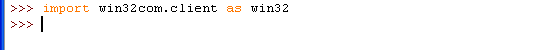If the import command was successful, you’ll see the “>>>” prompt returned. If there was a problem, such as not having the win32 module installed correctly, you’ll see `Import Error: Nomodule named win32com.client`. In that case, install the appropriate win32 module from the web site.

1. Start Excel

The command `win32.gencache.EnsureDispatch('Excel.Application')` attaches to an Excel process that is already running, or starts Excel if it’s not. If you see the “>>>” prompt, Excel has been started or linked successfully. At this point you won’t see Excel, but if you check your task manager you can confirm that the process is running.

1. Make Excel Visible

Setting the Visible flag with `excel.Visible = True` makes the Excel window appear. At this point, Excel does not contain any workbooks or worksheets, we’ll add those in the next step.

1. Add a workbook, select the sheet “Sheet1” and rename it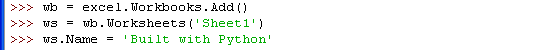Excel needs a workbook to serve as a container for the worksheets. A new workbook containing 3 sheets is added with command `wb = excel.Workbooks.Add()`. The command `ws =wb.Worksheets('Sheet1')` assigns `ws` to the sheet named Sheet1, and the command `ws.Name ='Built with Python'` changes the name of Sheet1 to “Built with Python”. Your screen should now look something like this: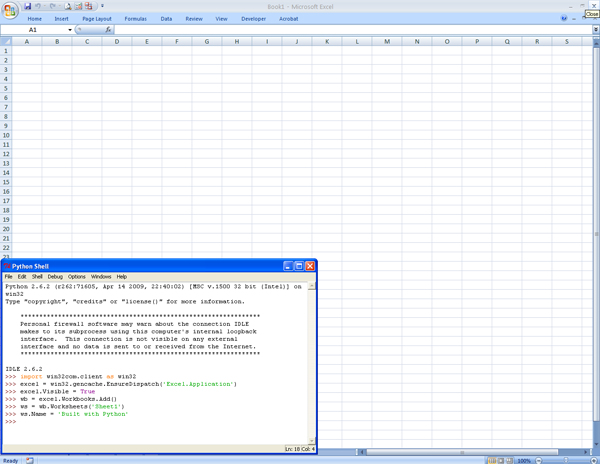1. Add some text into the first cell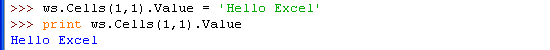Now the setup is complete and you can add data to the spreadsheet. There are several options for addressing cells and blocks of data in Excel, I’ll cover a few of them here. You can address individual cells with the `Cells(row,column).Value` pattern, where row and column are integer values representing the row and column location for the cell. Note that row and column counts begin from one, not zero. Use `.Value` to add text, numbers and date information to the cell and use `.Formula` for entering an Excel formula into the cell location.

After typing these commands, you’ll see the “Hello Excel” text in your Excel worksheet, and see the text printed in the IDLE window as well. Of course, Python can set values in the spreadsheet as well as query data from the spreadsheet.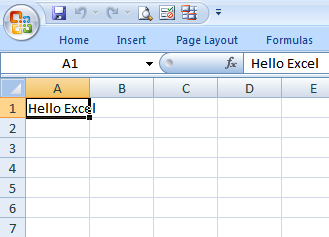1. Populate the second row with data by using a `for` loop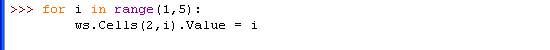In many cases you’ll have lists of data to insert into or extract from the worksheet. Wrapping the `Cells(row,column).Value` pattern with a loop seems like a natural approach, but in reality this maximizes the communication overhead between Python and Excel and results in very inefficient and slow code. It’s much better to transfer lists than individual elements whenever possible as shown in the next section. After this command, your Excel spreadsheet will look like this: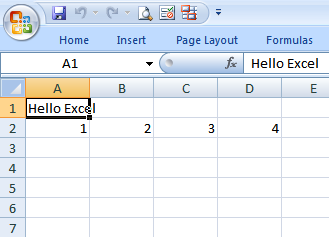1. Populate the third and fourth rows of data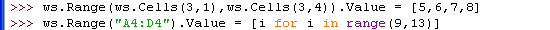A better approach to populating or extracting blocks of data is to use the `Range().Value` pattern. With this construct you can efficiently transfer a one- or two-dimensional blocks of data. In the first example, cells (3,1) through (3,4) are assigned to the list [5,6,7,8]. The next line uses the Excel-style cell address “A4:D4” to assign the results of the operation [`ifor i in range(9,13)]`. In some cases, it may be more intuitive to use the Excel-style naming. The Excel sheet now looks like this: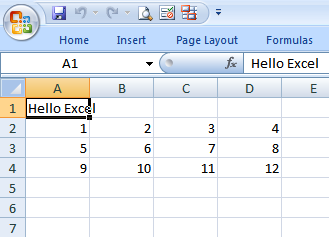1. Assign a formula to sum the numbers just added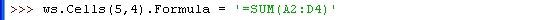You can insert Excel formulas into cells using the `.Formula` pattern. The formula is the same as if you were to enter it in Excel: `=SUM(A2:D4)`. In this example, the sum of 12 numbers in rows 2,3 and 4 is generated. Your Excel sheet should now look like the screenshot below.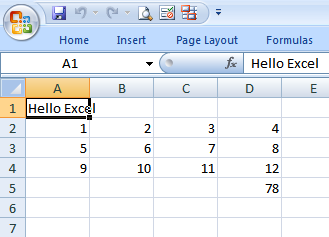1. Change the formatting of the formula cell **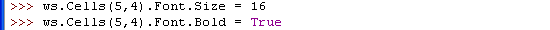As a final exercise, the format of the formula cell is changed to point size 16 with a bold typeface. You can change any of dozens of attributes for the various cells in the worksheet through Python. Your spreadsheet should now look like this.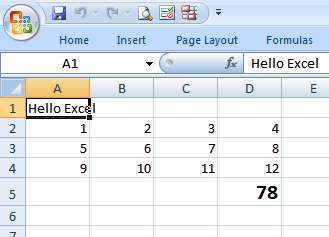Hopefully you did this exercise interactively, typing the commands and monitoring the result in Excel. You can also cut to the chase and run this script to generate the result. When the script exits, you’ll be left with an open Excel spreadsheet just as shown in the last screenshot above.

Prerequisites

Source Files and Scripts

Source for the program and data text file are available at http://github.com/pythonexcels/examples/tree/master

References

Core Python Programming

Wesley Chun’s book has a chapter on Programming Microsoft Office with Win32 COM